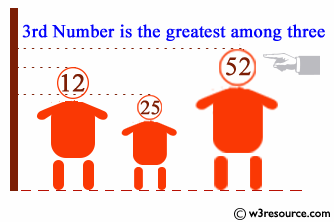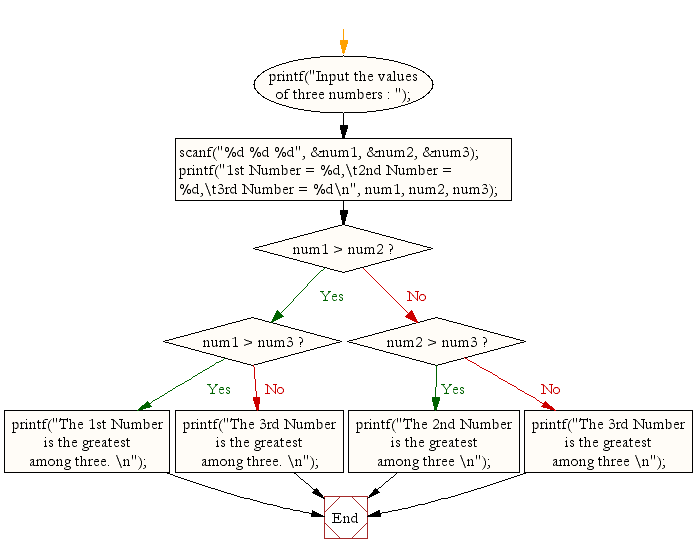﻿ C Program: Find the largest of three numbers - w3resource# C Exercises: Find the largest of three numbers

## C Conditional Statement: Exercise-8 with Solution

Write a C program to find the largest of three numbers.

Pictorial Presentation:Sample Solution:

C Code:

``````#include <stdio.h>
void main()
{
int num1, num2, num3;

printf("Input the values of three numbers : ");
scanf("%d %d %d", &num1, &num2, &num3);
printf("1st Number = %d,\t2nd Number = %d,\t3rd Number = %d\n", num1, num2, num3);
if (num1 > num2)
{
if (num1 > num3)
{
printf("The 1st Number is the greatest among three. \n");
}
else
{
printf("The 3rd Number is the greatest among three. \n");
}
}
else if (num2 > num3)
printf("The 2nd Number is the greatest among three \n");
else
printf("The 3rd Number is the greatest among three \n");
}
```
```

OR

``````#include <stdio.h>
void main() {
int num1, num2, num3;

printf("Input the values of three numbers : ");
scanf("%d %d %d", &num1, &num2, &num3);
printf("1st Number = %d,\t2nd Number = %d,\t3rd Number = %d\n", num1, num2, num3);

if ((num1 > num2) && (num1 > num3))
printf("The 1st Number is the greatest among three. \n");

if ((num2 > num3) && (num2 > num1))
printf("The 2nd Number is the greatest among three \n");

if ((num3 > num1) && (num3 > num2))
printf("The 3rd Number is the greatest among three. \n");

}
```
```

OR

``````#include <stdio.h>
void main() {
int num1, num2, num3,ctr;

printf("Input the values of three numbers : ");
scanf("%d %d %d", &num1, &num2, &num3);
printf("1st Number = %d,\t2nd Number = %d,\t3rd Number = %d\n", num1, num2, num3);

if (num1 > num2 && num1 > num3) ctr=num1;

if (num2 > num3 && num2 > num1) ctr=num2;

if (num3 > num1 && num3 > num2) ctr=num3;
printf("The %d is the greatest among three. \n",ctr);

}
```
```

Sample Output:

```Input the values of three numbers : 12 25 52
1st Number = 12,        2nd Number = 25,        3rd Number = 52
The 3rd Number is the greatest among three
```

Flowchart:C Programming Code Editor:

Improve this sample solution and post your code through Disqus.

What is the difficulty level of this exercise?

Test your Programming skills with w3resource's quiz.

﻿

## C Programming: Tips of the Day

Why do C and C++ compilers allow array lengths in function signatures when they're never enforced?

It is a quirk of the syntax for passing arrays to functions.

Actually it is not possible to pass an array in C. If you write syntax that looks like it should pass the array, what actually happens is that a pointer to the first element of the array is passed instead.

Since the pointer does not include any length information, the contents of your [] in the function formal parameter list are actually ignored.

Ref : https://bit.ly/3fhlvdH